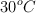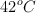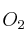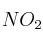# Ejercicios FyQ

Ejercicios Resueltos de Physics & Chemistry (3º ESO)

## Problemas y ejercicios en inglés para alumnos del programa bilingüe.

An element has atomic number 10 and atomic mass 20. How many neutrons does this element have?

Convert 7 678 ounces to pounds.

The volume of 200 g of a substance is 10 L, what is its density?

Calculate the final volume of a gas atand 1.1 atm, when its volume is 3 L and temperature and pressure are increased toand 1.3 atm.

The structure of a chemical compound is a cube. If the length of the edge of the cube is 500 pm, what will be the volume of the cube expressed in mL?

How many grams ofare needed to react with 120 g of NO, in order to obtain?

What are the units of mass and volume in SI? What will be the unit of density in SI?

The nucleus of an atom is composed of uncharged particles as well as of the following:

a) Electrons

b) Protons

c) Neutrons

d) Ions

Decide if these sentences are true or false and rewrite the false sentences correctly. Be careful because each mistake discounts a right answer:

a) Density of a solution is equal to the mass of the solute divided by the volume of the solvent.

b) The interaction between unlike charges is a repulsion force.

c) Distillation is the method to separate miscible liquids.

d) Decantation is the correct method to separate a mix of alcohol and water.

Índice de Ejercicios RESUELTOS

Buscar en esta web

Por palabras clave

Por temas

Búsqueda libre